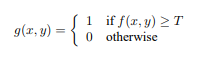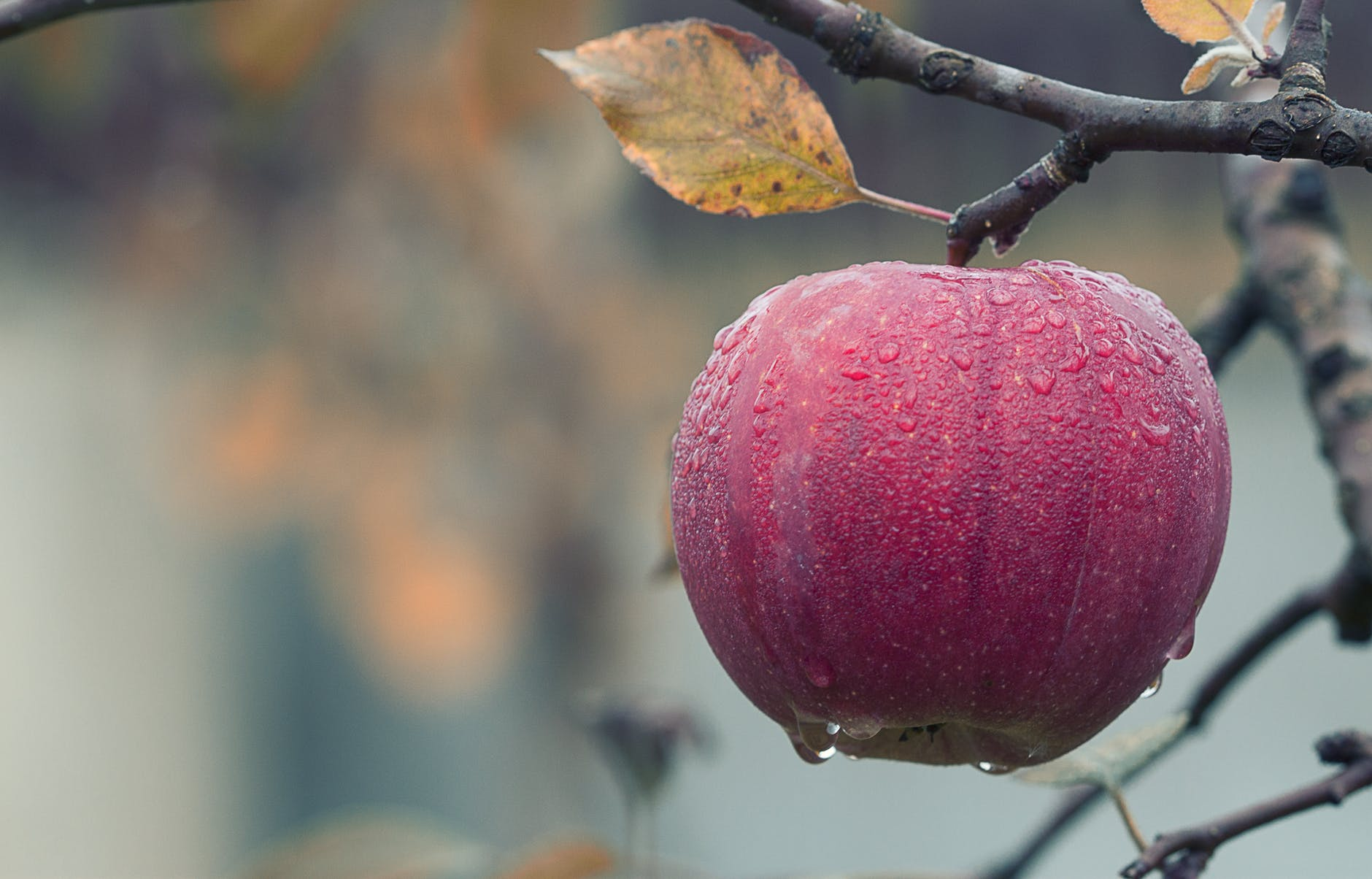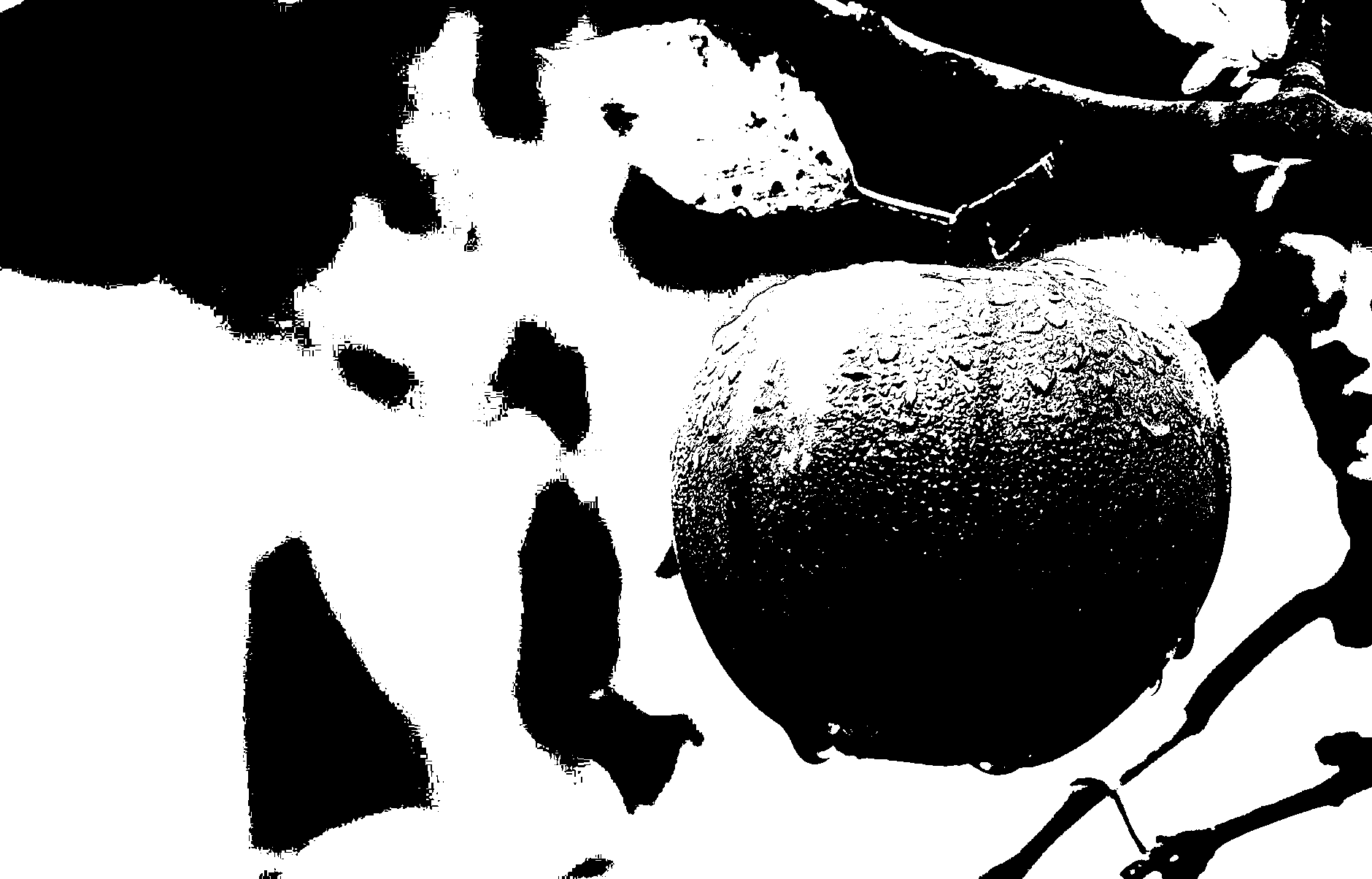Related Articles
MATLAB | Converting a Grayscale Image to Binary Image using Thresholding
• Difficulty Level : Basic
• Last Updated : 12 Sep, 2018

Thresholding is the simplest method of image segmentation and the most common way to convert a grayscale image to a binary image.

In thresholding, we select a threshold value and then all the gray level value which is below the selected threshold value is classified as 0(black i.e background ) and all the gray level which is equal to or greater than the threshold value are classified as 1(white i.e foreground).Here g(x, y) represents threshold image pixel at (x, y) and f(x, y) represents greyscale image pixel at (x, y).

Algorithm:

1. Read target image into MATLAB environment.
2. Convert it to a grayscale Image if read image is an RGB Image.
3. Calculate a threshold value, T
4. Create a new Image Array (say ‘binary’) with the same number of rows and columns as original image array, containing all elements as 0 (zero).
5. Assign 1 to binary(i, j), if gray level pixel at (i, j) is greater than or equal to the threshold value, T ; else assign 0 to binary(i, j).
Do the same for all gray level pixels.

Below is the implementation of above algorithm :

 `% Following MATLAB function will take a grayscale ``% or an RGB image as input and will return a``% binary image as output ``  ` `function` `[binary] = convert2binary(img) ``  ` `     ``[x, y, z]=size(img);``  ` `     ``% if Read Image is an RGB Image then convert ``     ``% it to a Gray Scale Image For an RGB image ``     ``% the value of z will be 3 and for a Grayscale``     ``% Image the value of z will be 1``  ` `    ``if` `z==3``         ``img=rgb2gray(img);``    ``end``  ` `    ``% change the class of image ``    ``% array from 'unit8' to 'double'``    ``img=double(img);``  ` `    ``% Calculate sum of all the gray level``    ``% pixel's value of the GraySacle Image``    ``sum=0;``    ``for` `i=1:x``         ``for` `j=1:y``        ``sum=sum+img(i, j);``     ``end``     ``end``  ` `    ``% Calculate Threshold value by dividing the ``    ``% calculated sum by total number of pixels ``    ``% total number of pixels = rows*columns (i.e x*y) ``    ``threshold=sum/(x*y);``   ` `    ``% Create a image array having same number``    ``% of rows and column as Original image``    ``% with all elements as 0 (Zero).``    ``binary=zeros(x, y);``  ` `    ``% iterate over all the pixels of Grayscale ``    ``% Image and Assign 1 to binary(i, j), if gray``    ``% level value is >=  threshold value     ``    ``% else assign 0 to binary(i, j) .``  ` `    ``for` `i=1:x``     ``for` `j=1:y``        ``if` `img(i, j) >= threshold``                ``binary(i, j) = 1;``        ``else``            ``binary(i, j)=0;``        ``end``     ``end``    ``end``end``  ` `  ` `% driver function ``  ` `% Read the target Image``img=imread(``'apple.png'``);``  ` `% Call convert2binary() function to convert``% Image to binary using thresholding``binary_image=convert2binary(img);``  ` `% Display result``imshow(binary_image);`

Input:Output: